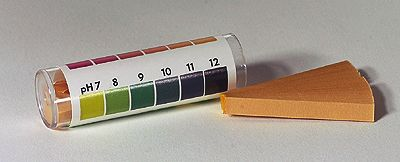PH Indicators And PH Paper

ChemistryWiki | RecentChanges | Preferences

What does a pH indicators do?
• Answer: As the name says, it indicates a pH. It does not determine if the solution is Acidic, Basic or Neutral.

How does it indicate a pH?
• At a specific pH, it changes from one color to another (or no color called colorless).

Why does a pH indicator changes colors?
• Every pH indicator (it is actually a weak acid, so it has a pKa) has an endpoint, and the color with change from the "acid form" color to the "base form" color as the solution pH goes by the endpoint (also a pH value). It is actually pH+ or - 1.0 units of pH. So the endpoint is actually a range not a point.

For example, the endpoint of the specific pH indicator is pH = 5.0, and the indicator changes from red ("acid form" color) to green ("base form" color). If I have a solution with this pH indicator in it and the initial pH is 2.0, then the solution is red. If I increase the pH, the solution will stay red until pH of 4.0 where it will start changing from a "pure" red to a mix of red and green until pH = 6.0 where the solution will be "pure" green. The solution will stay green for all pH above 6.0.

So, each pH indicator "changes" color at a different pH point (or more specifically range). So the pH indicator shows that the solution has "gone" thru that pH (called endpoint). As the following link shows most pH indicators (and their endpoints and the color changes) are not at a pH of 7.0. So not all pH indicator will determine if the solution is acidic (solution pH less than 7.0), neutral (solution pH of 7.0) or basic (solution pH of greater than 7.0). They will however, show if you are at a specific pH range.

Actual pH Indicator and pH paper

• Litmus paper (either blue or red paper) is the same thing. The pH indicator is litmus that is red in the "acidic form" and blue in the "basic form".

• Phenolphthalein - colorless in the "acidic form" and red in the "basic form". Endpoint is about pH=9.0. It is the most common pH indicator for titrations in 1st year high school chemistry class.
• pH paper (see below image) is actually a series of pH indicators that the combined colors will produce a single color so that every pH has a different color (comes with a pH vs. color scale).ChemistryWiki | RecentChanges | Preferences